Shipra Saxena — March 26, 2021

## Objective

• See the implementation of AdaBoostClassifer using python.

## Introduction

In the last article, we saw the Gradient boosting machine and how it works. It works on correcting the errors of previous models by building a new model on the error. The article on Gradient boosting Machine is available on the following link-

Now we will see another algorithm that uses the boosting technique, the Adaptive Boosting algorithm. It is more commonly known as AdaBoost.

Note: If you are more interested in learning concepts in an Audio-Visual format, We have this entire article explained in the video below. If not, you may continue reading.

In the case of AdaBoost, higher points are assigned to the data points which are miss-classified or incorrectly predicted by the previous model. This means each successive model will get a weighted input.

Let’s understand how this is done using an example.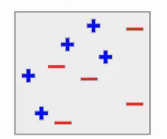Say, this is my complete data. Here, I have the blue positives and red negatives. Now the first step is to build a model to classify this data.

### Model1

Suppose the first model gives the following result, where it is able to classify two blue points on the left side and all red points correctly. But the model also miss-classify the three blue points here.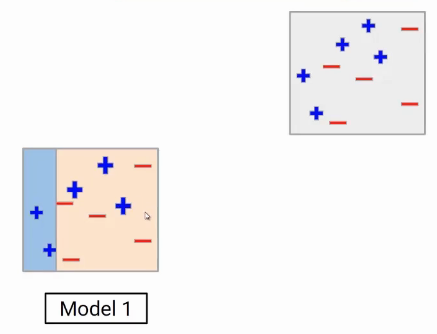### Model 2

Now, these miss- classified data points will be given higher weight. So these three blue positive points will be given higher weights in the next iteration. For representation, the points with higher weight are bigger than the others in the image. Giving higher weights to these points means my model is going to focus more on these values. Now we will build a new model.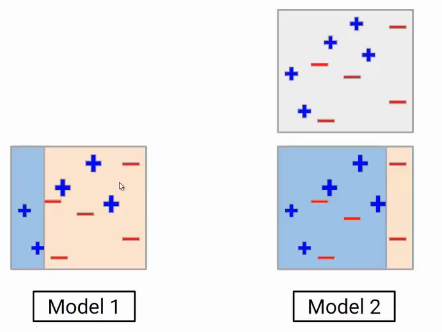In the second model you will see, the model boundary has been shifted to the right side in order to correctly classify the higher weighted points. Still, it’s not a perfect model. You will notice three red negatives are miss-classified by model 2.

### Model 3

Now, these miss-classified red points will get a higher weight. Again we will build another model and do the predictions. The task of the third model is two focus on these three red negative points. So the decision boundary will be something as shown here.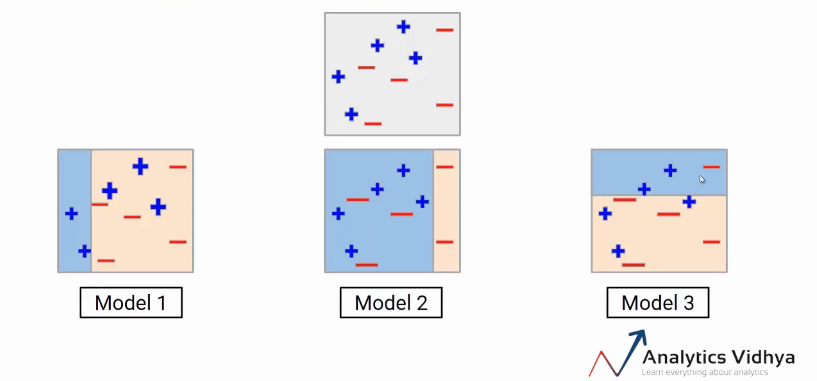This new model again incorrectly predicted some data points. At this point, we can say all these individual models are not strong enough to classify the points correctly and are often called weak learners.

### Ensemble

And guess what should be our next step. Well, we have to aggregate these models. One of the ways could be taking the weighted average of the individual weak learners. So our final model will be the weighted mean of individual models.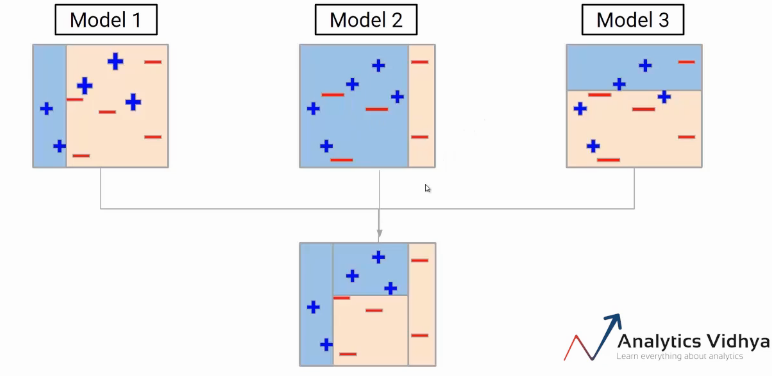After multiple iterations, we will be able to create the right decision boundary with the help of all the previous weak learners. As you can see the final model is able to classify all the points correctly. This final model is known as a strong learner.

Let’s once again see all the steps taken in AdaBoost.

1. Build a model and make predictions.
2. Assign higher weights to miss-classified points.
3. Build next model.
4. Repeat steps 3 and 4.
5. Make a final model using the weighted average of individual models.

## Implementation

Now we will see the implementation of the AdaBoost Algorithm on the Titanic dataset.

First, import the required libraries pandas and NumPy and read the data from a CSV file in a pandas data frame.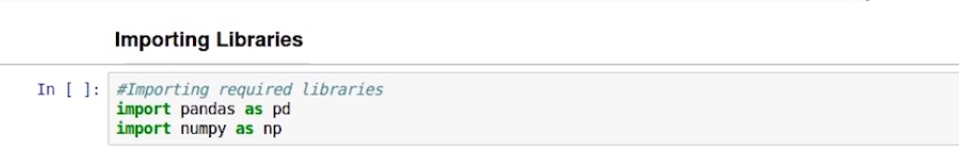Here are the first few rows of the data. Here we are using pre-processed data. So we can see it is a classification problem with those who were survived classified as 1 and those who not labeled as 0.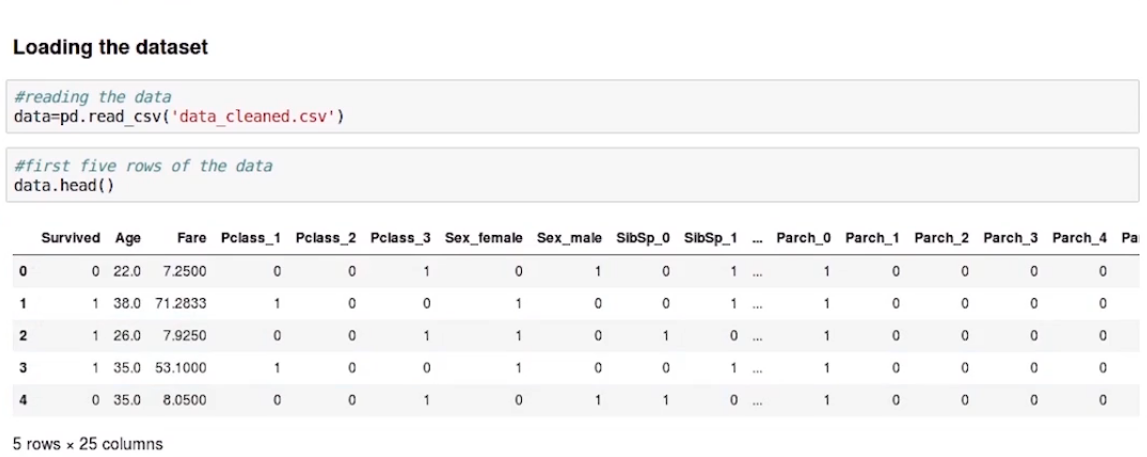In the next step, we will separate the independent and dependent variables, Saving the features in x and the target variable in y. Later, divide the data into train and test set using train_test split from sklearn. As shown below.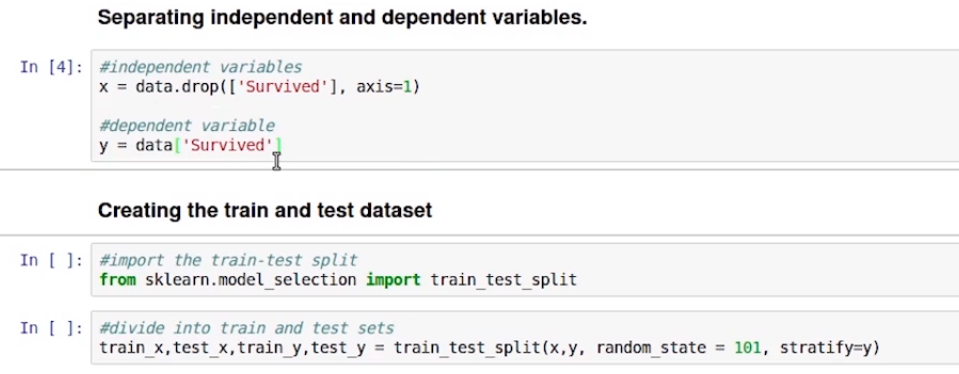Here the stratify is set to y, it is to make sure that the proportion of both the classes remain the same in both the train and test data. Say if you have 60% of class 1 and 40% of class 0 in train data then you would have the same distribution in the test.

Now we will import the AdaBoostClassifier from sklearn.ensemble and create an instance of the same. We have set the random state value to 96, to reproduce the result. Then we used train_x and train_y to train our model.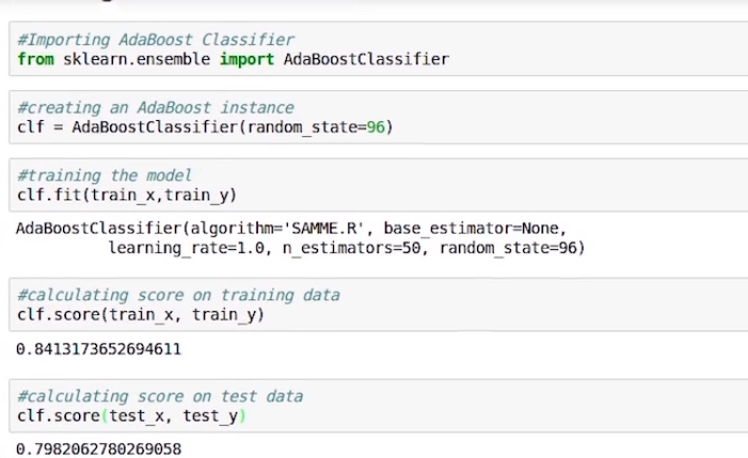Now let’s check the score on training data, it comes around .84. Now we will do the same for the test data and it comes out to be .79.

## Hyper-parameter tuning

Let’s have a look at the hyper-parameters of the AdaBoost model. Hyper-parameters are the values that we give to a model before we start the modeling process. Let’s see all of them.

base_estimator: The model to the ensemble, the default is a decision tree.

n_estimators: Number of models to be built.

learning_rate: shrinks the contribution of each classifier by this value.

random_state: The random number seed, so that the same random numbers generated every time.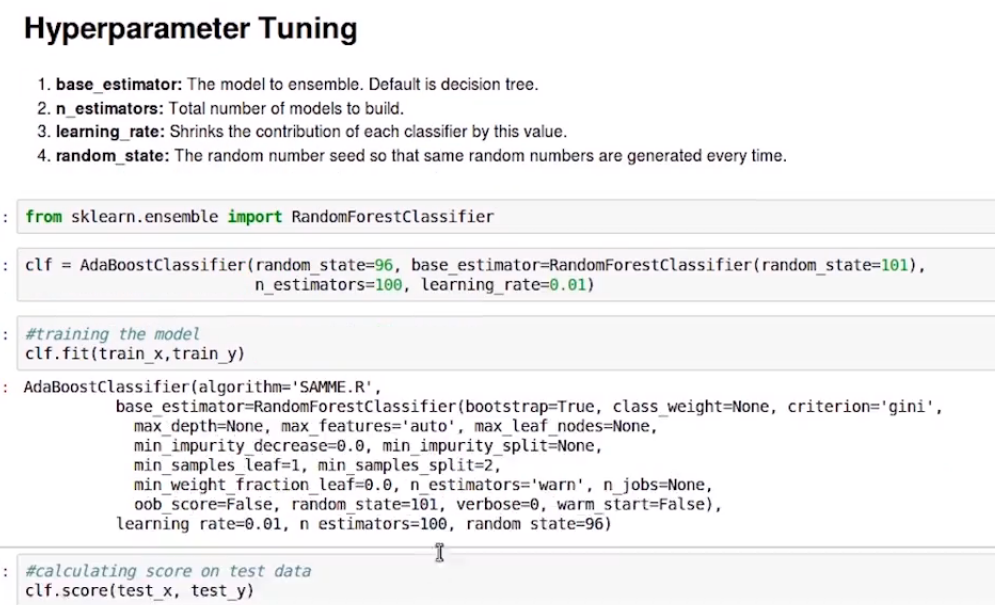To experiment with hyper-parameters, this time we have set the base_estimator  as RandomForestClassifier. We are using 100 estimators and learning_rate as 0.01. Now we train the model using the train_x and train_y as previously. Next is to check the score of the new model.

On the basis of the performance, you can change the hyper-parameters accordingly.

## End Notes

This was all about the AdaBoost algorithm in this article. Here we saw, how can we ensemble multiple weak learners to get a strong classifier. We also saw the implementation in python of the same.

If you are looking to kick start your Data Science Journey and want every topic under one roof, your search stops here. Check out Analytics Vidhya’s Certified AI & ML BlackBelt Plus Program

If you have any queries let me know in the comment section!